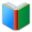Words for students of 8th Grade, the page is for math words (List 1), which are essential vocabulary for 8th Grade academic classes.
 Free Online Vocabulary TestK12, SAT, GRE, IELTS, TOEFL
 Actions upon current list

 All lists of current grdae
Spelling Words:

Arts Words:

Social Words:

Math Words:

Science Words:

Literature Words:Animal FarmThe Hound of the Baskervilles
 8th Grade Cards: Math (click the card to toggle it.)

(You can also click each card to show or hide its content.)
are angles that have a common ray coming out of the vertex going between two other rays, with no overlap of the regions
a constant number that serves as a measure of some property or characteristic
invariable; repeating; continually occurring; persistent
algebraic expression that gives the distance between two points in a particular coordinate system in a particular number of dimensions
field; territory over which rule or control is exercised; networked computers that share a common address
plan; directions for making something; a group of symbols that make a mathematical statement
the side of a right triangle opposite the right angle
the sum of the areas of the lateral (vertical) faces of a cylinder, cone, frustum, or the like
a polynomial equation of the first degree
an inequality which involves a linear function.
a relation in Euclidean geometry among the three sides of a right triangle, it states that the square of the hypotenuse is equal to the sum of the squares of the other two sides
the set of values of the dependent variable for which a function is defined
a type of display using Cartesian coordinates to display values for two variables for a set of data
a method of writing or displaying numbers in terms of a decimal number between 1 and 10 multiplied by a power of 10
be at an angle; incline; gradient
the equation of a straight line in the form y = mx b where m is the slope of the line and b is its y-intercept
geometric figure having three dimensions
the extent of a 2-dimensional surface enclosed within a boundary
limited period of time; point in time at which something ends; termination; deadline
 Quick Access Worksheet:
-->